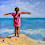# Arrays - Basic Java Tutorials For Selenium WebDriver

What is Array?
As we have learnt in my post about DIFFERENT DATA TYPES, We can store values in variables based on type of data like int i=5; double d = 12.254; etc in java software development. Now if you wants to store multiple values (10 or 15 or more different values) in different variables then it will be overhead for you to create and initialize different variables for each value. In this situation, you can use array to store multiple different values in array. An array can store multiple value of same data type(int, char, String) at the same time and each stored data location has unique Index. There are two types of array in java software development language. One Dimensional Array and Two Dimensional Array.

One Dimensional Array

Above given Image describes one dimensional array having one row of data. One dimensional array is just like spreadsheet with data in one row. As shown in above Image, Index of array Is starting from 0. Means value 10 has Index 0, 12 has Index 1 and so on and each value can be Identified by Its Index. We can create and Initialize values In array as shown In bellow given example.
``````public class Array_Example {

public static void main(String[] args) {
int a[] = new int; //Array declaration and Creation. 6 is length of array.
a = 10; //initialize 1st array element
a = 12; //initialize 2nd array element
a = 48; //initialize 3rd array element
a = 17; //initialize 4th array element
a = 5;  //initialize 5th array element
a = 49; //initialize 6th array element

for(int i=0; i<a.length; i++){
System.out.println(a[i]);
}
}

}``````

In above example, Length of array a[] is 6 and data type of array is int. Means we can store max 6 integer values in this array of java software development language. for loop helps to print value of each array cell. a.length will return length of array. When you will run above example in eclipse, You will get bellow given result in console.
``````a Holds 10
a Holds 12
a Holds 48
a Holds 17
a Holds 5
a Holds 49``````

Another way of creating and initializing same one dimensional array is as shown bellow.
``int a[] = {10,12,48,17,5,49};``

Two Dimensional Array

Tow dimensional array in java software development language is just like spreadsheet with multiple rows and columns having different data in each cell. Each cell will be Identified by it's unique row and column Index combination(Example str). We can use two dimensional array to store user id and password of different users as shown in above Image. For above given example Image, We can create and initialize values in two dimensional array as shown in bellow given example.
``````public class Twodimarray {

public static void main(String[] args) {
String str[][] = new String; //3 rows, 2 columns
str="User1";
str="User2";
str="User3";

for(int i=0; i<str.length; i++){//This for loop will be total executed 3 times.
for(int j=0; j<str[i].length; j++){//This for loop will be executed for 2 time on every iteration.
System.out.println(str[i][j]);
}
}
}
}``````

When you will run this example in eclipse, It will return bellow given result in console.
``````User1
User2
User3

Other way of creating and initializing same two dimensional array is as shown bellow.
``String str[][] = {{"User1","Password1"},{"User2","Password2"},{"User3","Password3"}};``

1.Explained very well

2.3.I am not understanding this part. I have read the tutorial on For loops and thought i understood it. Can someone please explain what 'i' and 'j' stand for and what determines that the loop for 'i' will be iterated 3 times but the loop for 'j' will be looped for only two times?

for(int i=0; i<str.length; i++){//This for loop will be total executed 3 times.
for(int j=0; j<str[i].length; j++){//This for loop will be executed for 2 time on every iteration.

4.Here i and j are indexes. Above example is a two dimensional array of 3 rows and 2 columns. In order to iterate through all 3 rows the loops executes 3 times and for 2 columns it iterates 2 times.

for an array,
array--row0column0--user1

array--row1column0--user2

array--row2column0--user3

5.What value return in str[i].length syntax?

1.str[i].length will return 2

Because in above example,

str[i] represents each row and each row length i.e., str.length, str.length, str.length is 2.

Hope this helps. Please correct if my understanding is wrong.

2.Thanks u very much.

6.Thanks u very much.

7.the result shown is not two dimensional:(

if we rewrite like
for(int i=0; i<str.length; i++)
{
for(int j=0; j<str[i].length; j++)
{
System.out.print(str[i][j]);
}
System.println(" ")
}
result in the console will be in 2 dimensional array

8.Aravind G! You are a blessing for learners!

9.How can we pass the array into a webdriver selector?
For eg. String str[] = { "Option1", "Option2" };
driver.findElement(By.cssSelector(str[])).click();

Will that be possible?

1.@anowar yes you can do that but like this

driver.findElement(By.cssSelector(str)).click();

instead of the thing you pass in " " you can simply write str if its single dimension if its multi dimensional then you can write str